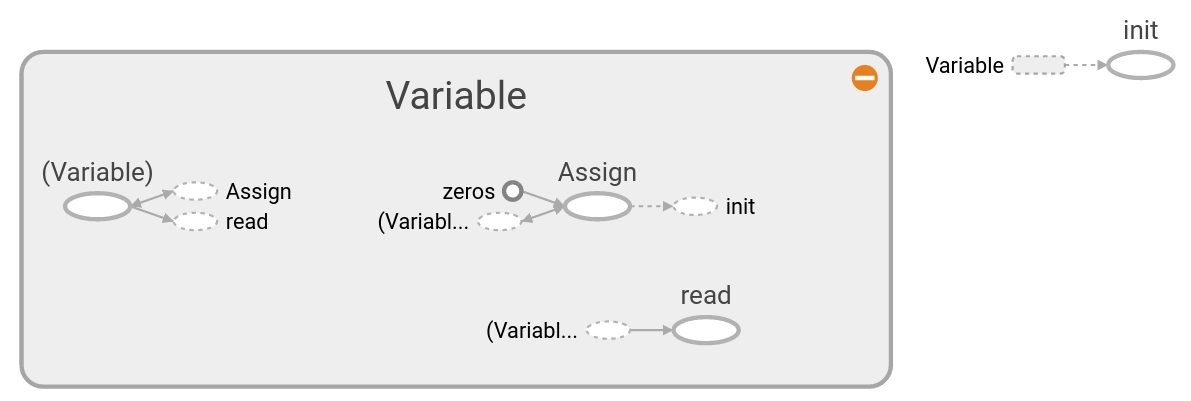# 创建变量和占位符¶

```>>> my_var = tf.Variable(tf.zeros([1,20]))
>>> sess.run(my_var)
Traceback (most recent call last):
...
FailedPreconditionError: 2 root error(s) found.
```

```>>> sess.run(my_var.initializer)
>>> sess.run(my_var)
array([[ 0.,  0.,  0.,  0.,  0.,  0.,  0.,  0.,  0.,  0.,  0.,  0.,  0.,
0.,  0.,  0.,  0.,  0.,  0.,  0.]], dtype=float32)
```

```>>> my_var1 = tf.Variable(tf.zeros([2,3]))
>>> sess = tf.compat.v1.Session()
# 初始化全局变量
>>> initialize_op = tf.compat.v1.global_variables_initializer()
>>> sess.run(initialize_op)
```

```>>> import numpy as np
>>> sess = tf.compat.v1.Session()
>>> x = tf.compat.v1.placeholder(tf.float32,shape=[2,2])
>>> y = tf.compat.v1.identity(x)
>>> x_vals = np.random.rand(2,2)
>>> sess.run(y, feed_dict={x: x_vals})
array([[0.8200612 , 0.53398275],
[0.5647656 , 0.84022015]], dtype=float32)

>>> sess.run(x,feed_dict={x: x_vals})
array([[0.8200612 , 0.53398275],
[0.5647656 , 0.84022015]], dtype=float32)
```

```>>> initializer_op = tf.compat.v1.global_variables_initializer()
```

```>>> sess = tf.compat.v1.Session()
>>> first_var = tf.Variable(tf.zeros([2,3]))
>>> sess.run(first_var.initializer)
# 取决于第一个变量
>>> second_var = tf.Variable(tf.zeros_like(first_var))
>>> sess.run(second_var.initializer)
```

# 创建特定的变量¶

```>>> row_dim = 2
>>> col_dim = 3
```

```>>> zero_var = tf.Variable(tf.zeros([row_dim, col_dim]))
>>> ones_var = tf.Variable(tf.ones([row_dim, col_dim]))
```

```>>> sess.run(zero_var.initializer)
>>> sess.run(ones_var.initializer)
>>> print(sess.run(zero_var))
[[ 0.  0.  0.]
[ 0.  0.  0.]]
>>> print(sess.run(ones_var))
[[ 1.  1.  1.]
[ 1.  1.  1.]]
```

# 基于其他张量的形状创建张量¶

```>>> zero_similar = tf.Variable(tf.zeros_like(zero_var))
>>> ones_similar = tf.Variable(tf.ones_like(ones_var))
>>> sess.run(ones_similar.initializer)
>>> sess.run(zero_similar.initializer)
>>> print(sess.run(ones_similar))
[[ 1.  1.  1.]
[ 1.  1.  1.]]
>>> print(sess.run(zero_similar))
[[ 0.  0.  0.]
[ 0.  0.  0.]]
```

# 常数填充变量张量¶

```>>> fill_var = tf.Variable(tf.fill([row_dim, col_dim], -1))
>>> sess.run(fill_var.initializer)
>>> print(sess.run(fill_var))
[[-1 -1 -1]
[-1 -1 -1]]
```

```# 通过常数列表来创建张量
>>> const_var = tf.Variable(tf.constant([8, 6, 7, 5, 3, 0, 9]))
# 通过常数数组来创建变量张量
>>> const_fill_var = tf.Variable(tf.constant(-1, shape=[row_dim, col_dim]))

>>> sess.run(const_var.initializer)
>>> sess.run(const_fill_var.initializer)

>>> print(sess.run(const_var))
[8 6 7 5 3 0 9]
>>> print(sess.run(const_fill_var))
[[-1 -1 -1]
[-1 -1 -1]]
```

# 基于序列和range来创建变量张量¶

```# TensorFlow的中linspace
>>> linear_var = tf.Variable(tf.linspace(start=0.0, stop=1.0, num=3))
# Generates [0.0, 0.5, 1.0] includes the end

# TensorFlow的range
>>> sequence_var = tf.Variable(tf.range(start=6, limit=15, delta=3))
# Generates [6, 9, 12] doesn't include the end

>>> sess.run(linear_var.initializer)
>>> sess.run(sequence_var.initializer)

>>> print(sess.run(linear_var))
[ 0.   0.5  1. ]
>>> print(sess.run(sequence_var))
[6  9 12]
```

# 随机数变量张量¶

```>>> rnorm_var = tf.compat.v1.random_normal([row_dim, col_dim], mean=0.0, stddev=1.0)
>>> runif_var = tf.compat.v1.random_uniform([row_dim, col_dim], minval=0, maxval=4)

>>> print(sess.run(rnorm_var))
[[ 1.1772728   1.36544371 -0.89566803]
[-0.02099477 -0.17081328  0.2029814 ]]
>>> print(sess.run(runif_var))
[[ 2.54200077  1.42822504  1.34831095]
[ 2.28473616  0.36273813  0.70220995]]
```

# 在TensorBoard中进行变量创建的可视化¶

```# 重设计算图
>>> ops.reset_default_graph()

# 开始一个graph session
>>> sess = tf.compat.v1.Session()

# 创建变量张量
>>> my_var = tf.Variable(tf.zeros([1,20]))

# 将summary加到Tensorboard上
>>> merged = tf.compat.v1.summary.merge_all()

# 初始化图形写入
>>> writer = tf.compat.v1.summary.FileWriter("/tmp/variable_logs", graph=sess.graph)

# 全局变量初始器
>>> initialize_op = tf.compat.v1.global_variables_initializer()

# 变量初始化
>>> sess.run(initialize_op)
```

```\$ tensorboard --logdir=/tmp
```